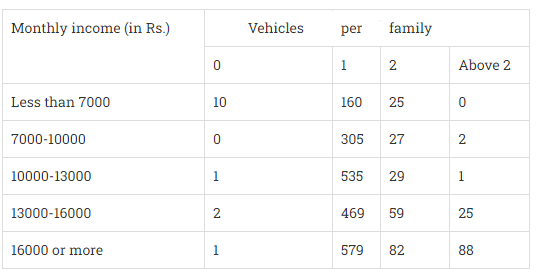# An organisation selected 2400 families at random and surveyed them to determine arelationship between income level and the number of vehicles in a family. The information gathered is listed in the table below:Suppose a family is chosen. Find the probability that the family chosen is(i) earning $Rs. 10000-13000$ per month and owning exactly 2 vehicles.(ii) earning $Rs. 16000$ or more per month and owning exactly 1 vehicle.(iii) earning less than Rs. 7000 per month and does not own any vehicle.(iv) earning $Rs. 13000-16000$ per month and owning more than 2 vehicles.(v) owning not more than 1 vehicle."

Given:

An organisation selected 2400 families at random and surveyed them to determine a relationship between income level and the number of vehicles in a family.

A family is chosen.

To do:

We have to find the probability that the family chosen is

(i) earning $Rs. 10000-13000$ per month and owning exactly 2 vehicles.
(ii) earning $Rs. 16000$ or more per month and owning exactly 1 vehicle.
(iii) earning less than Rs. 7000 per month and does not own any vehicle.
(iv) earning $Rs. 13000-16000$ per month and owning more than 2 vehicles.
(v) owning not more than 1 vehicle.

Solution:

(i) Total number of families $=2400$

Number of families earning Rs 10000-13000 per month and owning exactly 2 vehicles $=29$

We know that,

Probability of an event=$\frac{Number \ of \ favourable \ outcomes}{Total \ number \ of \ outcomes}$

Therefore,

Probability that the family is earning Rs 10000-13000 per month and owning exactly 2 vehicles $=\frac{29}{2400}$

The probability the family is earning Rs 10000-13000 per month and owning exactly 2 vehicles is $\frac{29}{2400}$.

(ii) Total number of families $=2400$

Number of families earning Rs 16000 or more per month and owning exactly 1 vehicle $=579$

We know that,

Probability of an event=$\frac{Number \ of \ favourable \ outcomes}{Total \ number \ of \ outcomes}$

Therefore,

Probability that the family is earning Rs 16000 or more per month and owning exactly 1 vehicle $=\frac{579}{2400}$

The probability the family is earning Rs 16000 or more per month and owning exactly 1 vehicle is $\frac{579}{2400}$.

(iii) Total number of families $=2400$

Number of families earning less than Rs 7000 per month and does not own any vehicle $=10$

We know that,

Probability of an event=$\frac{Number \ of \ favourable \ outcomes}{Total \ number \ of \ outcomes}$

Therefore,

Probability that the family is earning less than Rs 7000 per month and does not own any vehicle $=\frac{10}{2400}$

$=\frac{1}{240}$

The probability the family is earning less than Rs 7000 per month and does not own any vehicle is $\frac{1}{240}$.

(iv) Total number of families $=2400$

Number of families earning Rs 13000-16000 per month and owning more than 2 vehicles $=25$

We know that,

Probability of an event=$\frac{Number \ of \ favourable \ outcomes}{Total \ number \ of \ outcomes}$

Therefore,

Probability that the family is earning Rs 13000-16000 per month and owning more than 2 vehicles $=\frac{25}{2400}$

$=\frac{1}{96}$

The probability the family is earning Rs 13000-16000 per month and owning more than 2 vehicles is $\frac{1}{96}$.

(v) Total number of families $=2400$

Number of families owning not more than 1 vehicle $=10 + 0 + 1 + 2 + 1 + 160 + 305 + 535 + 469 + 579=2062$

We know that,

Probability of an event=$\frac{Number \ of \ favourable \ outcomes}{Total \ number \ of \ outcomes}$

Therefore,

Probability that the family is owning not more than 1 vehicle $=\frac{2062}{2400}$

$=\frac{1031}{1200}$

The probability the family is owning not more than 1 vehicle is $\frac{1031}{1200}$.